Rotational Symmetry

Chapter 14 Class 7 Symmetry
Concept wise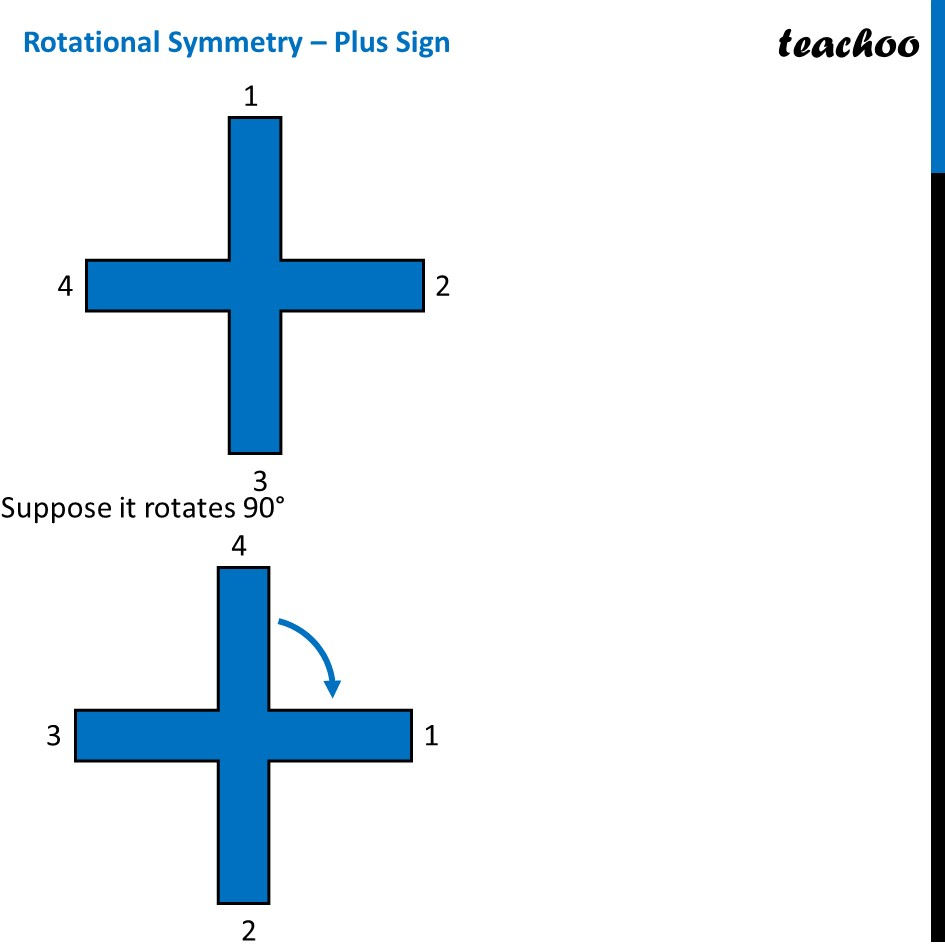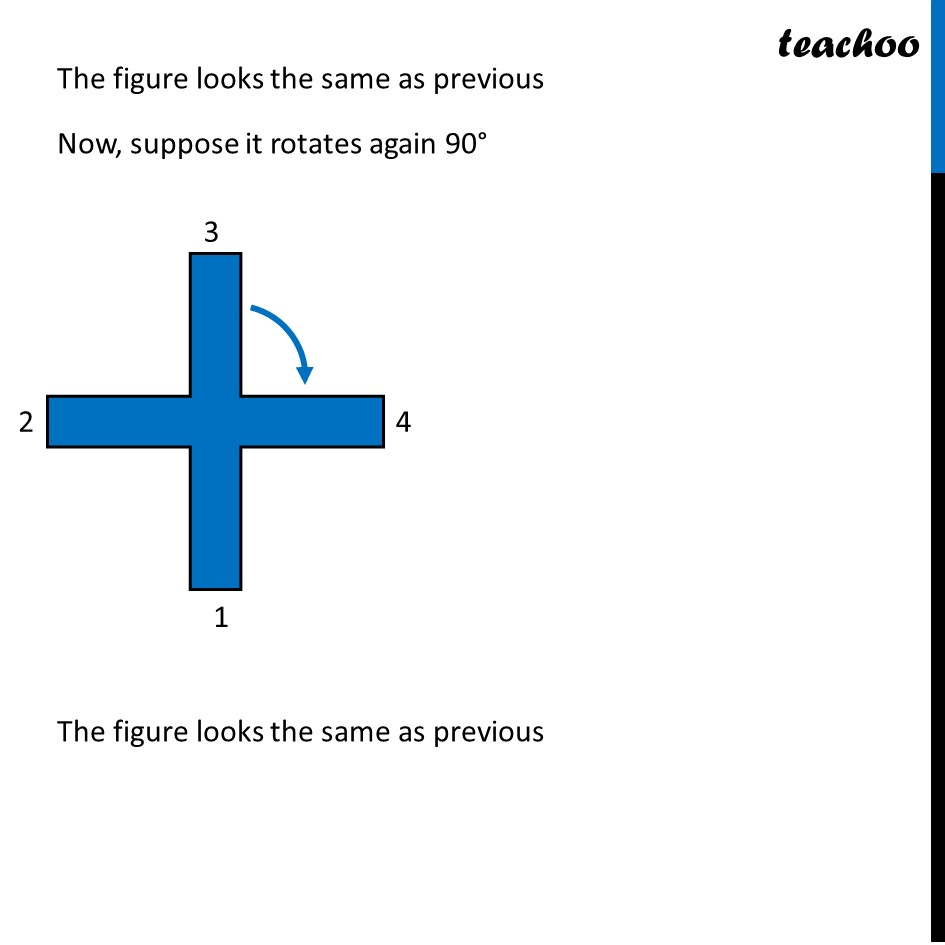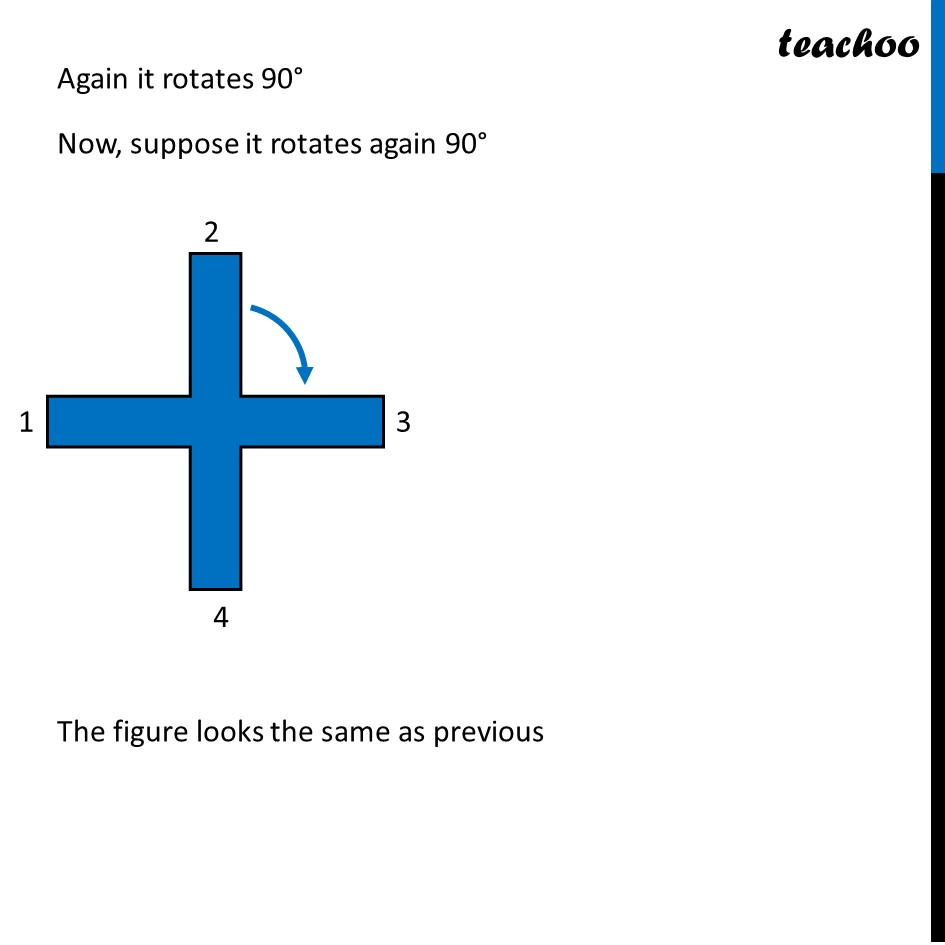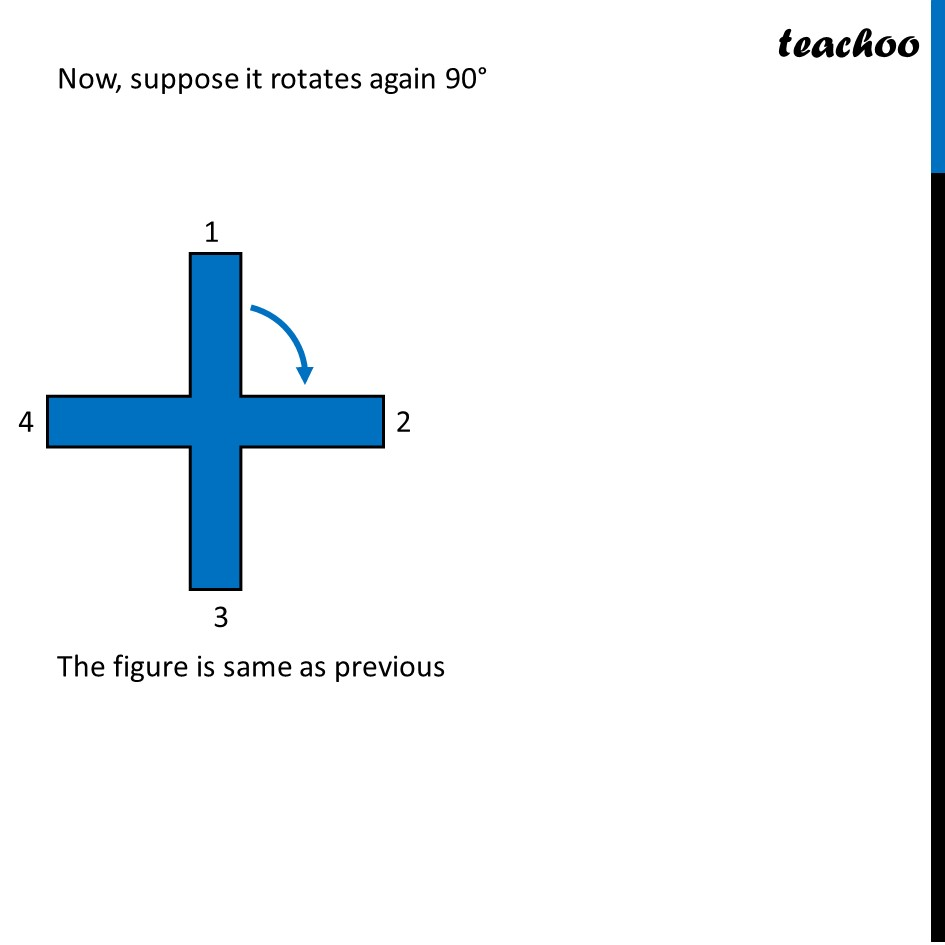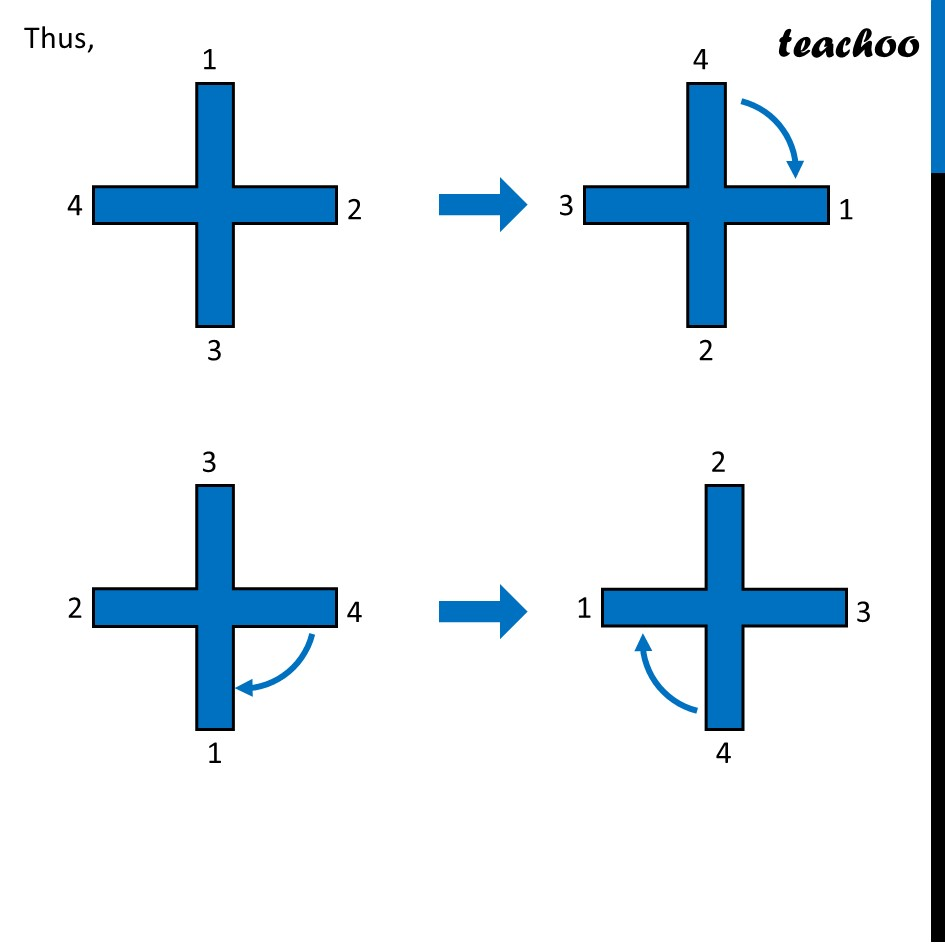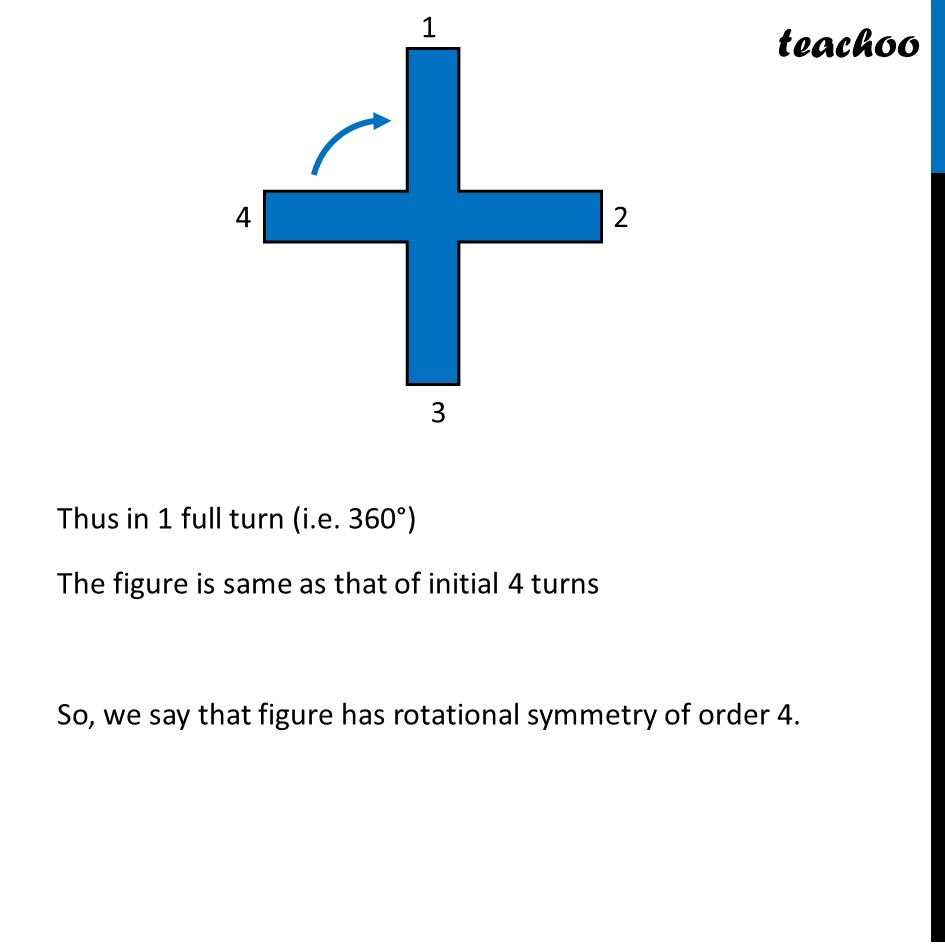### Transcript

Rotational Symmetry – Plus SignSuppose it rotates 90° The figure looks the same as previous Now, suppose it rotates again 90° The figure looks the same as previous Again it rotates 90° Now, suppose it rotates again 90° The figure looks the same as previous Now, suppose it rotates again 90° The figure is same as previous Thus, Thus in 1 full turn (i.e. 360°) The figure is same as that of initial 4 turns So, we say that figure has rotational symmetry of order 4.